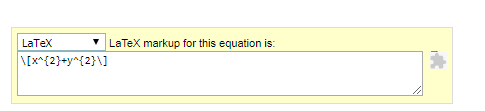Adding Maths equations to WordPress without any plugin is possible and it will also not render them as images. If you are running an educational website that primarily focused on the subjects like Physics, Chemistry, Mathematics or any other Engineering subject then it is very necessary to your website to support the inline equations (between your content line or anywhere).

Here you will get easiest way to add the maths equations to WordPress without any plugin as well as not as the images by following the steps given below:

<script type="text/javascript" src="https://cdn.mathjax.org/mathjax/latest/MathJax.js?config=TeX-AMS-MML_HTMLorMML">
</script>

2. Goto the https://www.codecogs.com/latex/eqneditor.php

3. Make the equation that you want to insert in the latex form.

4. Now Scroll down and select the Latex from the drop-down menu and copy the equation code.5. Paste the equation code where you want to publish on your website.

Note: If you want to publish the equation as inline equation simply replace the $equation here$ to the $$equation here$$.

This is the best way to show maths equations from the latex code. There are many other alternatives of showing the mathematical equations but we found this one is best among all. As it will directly render the equation from the latex. There are many plugins available that render the equations as image and too many images on your website can reduce the Google Page Speed Score.

If you are not comfortable and don’t know how to add the codes or want short training then you can contact us via Comment or via WhatsApp to 8755077650.

If you are running Wp Pro Quiz on your website then check the latest features customized by us – Wp Pro Quiz New Features.